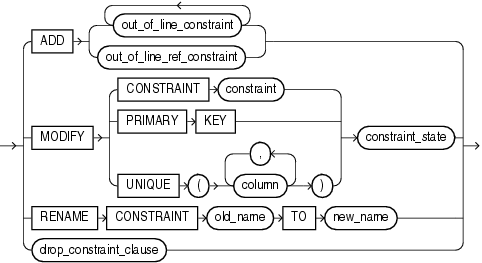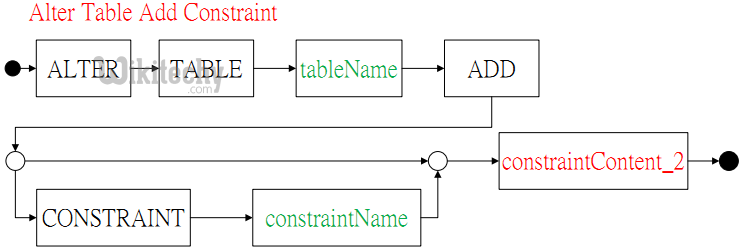# Add Constraint SQL - sql - sql tutorial - learn sql• SQL Constraints are rules, which is used to limit the type of data that can go into a table.
• To maintain the accuracy & integrity of the data within the table.
• Constraints are used to make sure that the integrity of data is maintained in the database.
• Constraints can be divided into two types as follows,

## Column level constraints :

• limits only column data

## Table level constraints :

• limits whole table data
• Following are the most used constraints that can be applied to a table.
• Not null
• Unique
• Primary key
• Foreign key
• Check
• Default

## SQL > ALTER TABLE > Add Constraint Syntax

• Sometimes we may decide to add a new constraint to an existing table (to see what are the different types of constraints that can be located on a database table, ref. to the CONSTRAINT section).• The syntax for adding a constraint in SQL is,
• For example, assuming our initial point is the Employee table created in the CREATE TABLE section:

## Table Employee

Column Name Data Type
First_Name char(50)
Last_Name char(50)
City char(50)
Country char(25)
Birth_Date datetime
• Assume we want to add a UNIQUE constraint to the "Address" column. To do this, we type in the following:

## SQL Server:

• where Wikitechy is the name of the constraint.

## Alter Table Add Constraint